# Introduction

## Background and Motivation

MatrixPlot is a program to display high quality matrix plots of any type of data given in a simple format, the .mp format. MatrixPlot have a number of options to tune the plot according to specifications given by the user. In particular the user may display additional information along the edges of the plot and zoom in on any region of the plot. For further introduction consult the MatrixPlot paper
MatrixPlot: a program to visualize sequence constraints. J. Gorodkin, H. H. Stærfeldt, O. Lund, and S. Brunak. Bioinformatics. 15:769-770, 1999. (http://www.cbs.dtu.dk/services/MatrixPlot/)
MatrixPlot is accompanied by two other programs: Inform, which computes the mutual information between any two positions in a sequence alignment, and produces the output in the mp format. The second program pdb2mp takes a PDB file from Brookhaven Protein Data Bank and computes all the interatomic distance between either the C-alpha atoms in proteins or P atoms in nucleotide sequences. The output is produced in the mp format. The modularity of the program structure is simple:In particular for the user program, one can export the output of any web server directly to MatrixPlot by using hidden variables in a submission form. The zoom program cuts out a desired region of the matrix plot. It can be reused arbitrarily many times on the mp file typically after consulting the output from MatrixPlot.
The mutual information content of a structural alignment (refound by foldalign of the following RNA sequences (sequences from Tuerk et al. PNAS 89, pp 6988-6992, 1992.):
 CCAGAGGCCCAACUGGUAAACGGGC CCG-AAGCUCAACGGGAUAAUGAGC CCG-AAGCCGAACGGGAAAACCGGC CC-CAAGCGC-AGGGGAGAA-GCGC CCG-ACGCCA-ACGGGAGAA-UGGC CCGUUUUCAG-UCGGGAAAAACUGA CCGUUACUCC-UCGGGAUAAAGGAG CCGUAAGAGG-ACGGGAUAAACCUC CCG-UAGGAG-GCGGGAUAU-CUCC CCG--UGCCG-GCGGGAUAU-CGGC CCG-AACUCG-ACGGGAUAA-CGAG CCG--ACUCG--CGGGAUAA-CGAG
can be displayed by MatrixPlot as: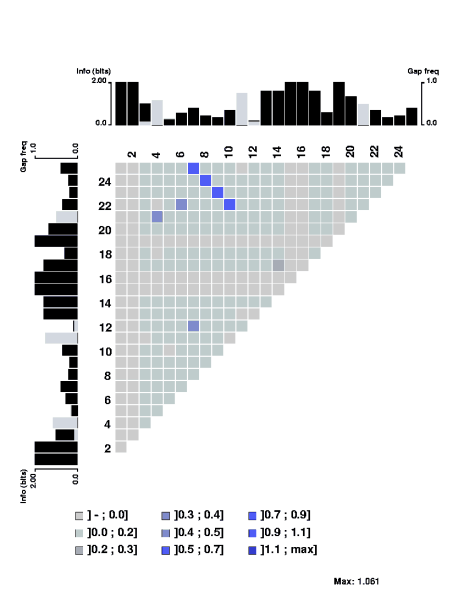The degree of mutual information is indicated by the color scale. Below we discuss convenient ways to compute the mutual information content of such a structural alignment. Along the edges the plain sequence information content along with gap frequencies are displayed. The scale to the left of the profile indicates the sequence information, which is plotted in black. The scale to the right indicates the frequency of gaps. The sequence information is computed as in the structure logo, but when including gaps we have introduced displacement of the baseline of the plain sequence information content profile. This is discussed below. If, at some position in the alignment, the gap frequency uses a larger bar than than for the sequence information content, the information bar is displayed on top of the gap bar, and vice versa.
For sequences with a set of three-dimensional coordinates, for example PDB entry 1raa chain A, MatrixPlot generates the plot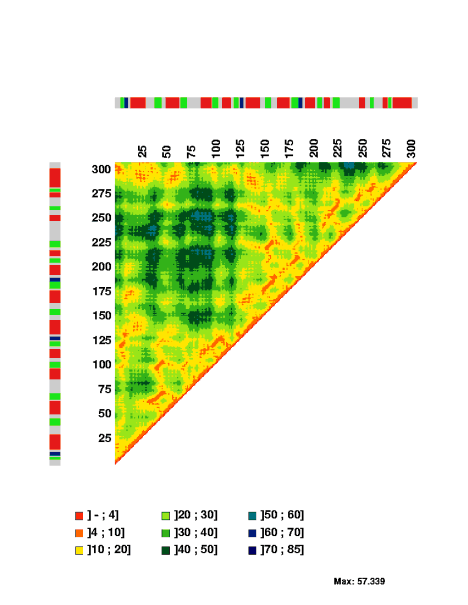The interatomic distances between the C-alpha atoms are indicated by the colorscales, which measures distances in Angstrom. The colors in the bar express the secondary structure assignment for the PDB file: helix is indicated in red; sheet is indicated in green; turn is indicated in blue. The color gray indicate residues without assignment in the PDB file.
Below it is discussed how to modify the graphical output of MatrixPlot. The graphical output is given in postscript files. Any submitted data is kept confidential and deleted a short time after submission.

## Program Options (man pages)

The options to Inform, pdb2mp, MatrixPlot, and Zoom are described in the pages given below. Source code requests: send email to gorodkin@cbs.dtu.dk.

## WWW Interface

The web interface of MatrixPlot consist of four pages, and has been made from the programs presented above: mutual information plots for RNA or DNA alignments, mutual information plots for protein alignments, distance matrices of sequences with a set of three-dimensional coordinates, and a page that takes a user produced mp file. Some of the command line options of the individual programs have been combined into one field on the corresponding web page. This is described here:
• Mutual information for nucleotide sequence alignments
This page contains the options to Inform and all the relevant options of MatrixPlot to generate mutual information plots.
• Mutual information for protein sequence alignments
This page contains the options to Inform ("complementarity matrix" is not defined) with mtype=1 (standard form of mutual information), and all the relevant options of MatrixPlot.
• Distance matrices
This page contains the options to pdb2mp, as well as the relevant MatrixPlot options to generate distance matrices of sequences with a set of 3D coordinates. The user may also enter the name of a PDB entry. If the chain identifier is omitted a distance matrix will be generated for the first identifier in the PDB entry.
• User matrix
This page allows the user to submit an mp file. All MatrixPlot options are available on that page.
After submission to the web pages, the matrix plot is returned as gif and postscript files. The colors on the gif file are limited, so they might be distorded depending on the browser. Along with the matrix plots a new form is given. This form includes all the previous MatrixPlot settings as well as options to zoom around in the plot. Upon resubmission new matrix plots are returned together with a new submission form. The following two plots illustrate this. First a full matrix plot of the archaea RNA sequences downloaded from the Signal recognition particle database (Samuelsson and Zwieb, Nucl. Acids Res. 27 pp 169-170, 1999). The trace of lines from upper left corner towards lower right corner indicate strecthes of covariant positions in the alignment.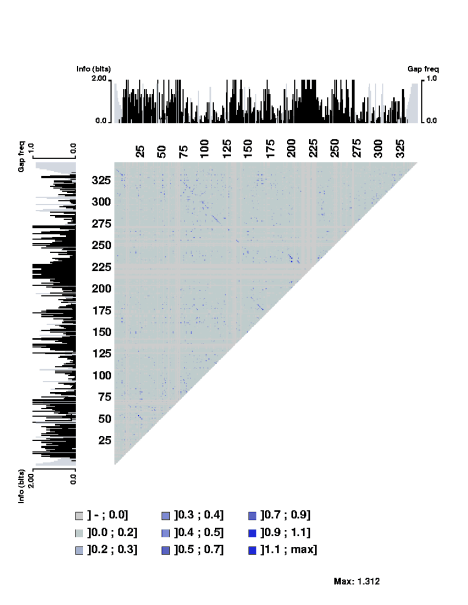Then using the zoom option result in the following plot: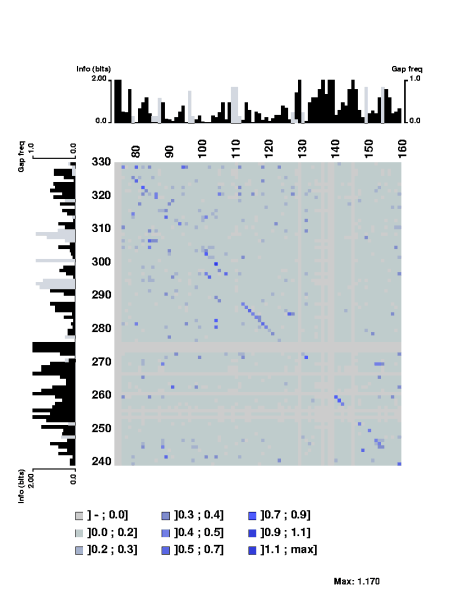The stretch of covarying positions corresponds to the main stem of the RNA secondary structure. Note that the trace is interrupted by a few positions having complete sequence conservation. The respective parts of the sequence logo profile have also been displayed along the edges of the plot.

Export user data directly to MatrixPlot
The user data can automatically be exported to MatrixPlot thorugh the web. This would typically be data generated from another web page which then can be exported for graphical manipulation, such as zooming in on a region. Only a single button is needed, as the form can be written using hidden variables.

## Discussion of Information Content

Two issues on information content for sequence/structure alignments are discussed here. First the contribution to the computation of information content when gaps are included in the alignment, and secondly the sample size robustness of mutual information for RNA secondary structure co-variance analysis. For background and details consult the mentioned references.

• Calculating the information content of an alignment that have gaps.

The conceptual problem in computing the information content or relative entropy of an alignment that has gaps is the background probablities. The background probabilities are typically found by counting base (or amino acid) frequencies in the genomes (or data set) from which the aligned sequences originate. The gaps, however, do not appear before the alignment is made, so a ``gap background probability'' or a ``gap expectation value'' cannot be dealt with in the same way. It only makes sense to talk about this when the alignment has been performed.

Calculation of the information content of an alignment containing gaps has been derived by Hertz and Stormo, 1995 (In Lim & Cantor (eds), Proc. of the Third Int. Conf. on Bioinformatics and genome Res., pp. 201-216). They derived the expressionwhere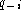andare the fraction of gaps and symbol k at position i in the alignment, and where k is a symbol in the alphabet A. The fractionis the background probability of symbol k, so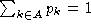. Note that if only a few sequences contain a gap (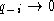) or almost all sequences have a gap () at some position i, the ``gap'' term does not contribute to the sum. Since the gap background probability is one in this expression, then all the background probabilities sum to 2 rather than one, which is the formal claim to define information content or relative entropy. This is resolved as follows. Hertz and Stormo derived a large-devation rate function given byand as they write, it normalizes all background probabilities with a factor of 1/2. This corresponds to addingto the information content on each of L positions in the alignment. Clearly, the difference between I and, is where to put the baseline in a sequence logo profile plot. The normalizing factor of Hertz and Stormo was found by considering the minimum of. When using the normalizing factor, one interpretation is that the a priori expectation to gaps is probability 0.5, however, another interpretation is that one has two models, one for which the expectation values for all the symbols sum to one, and one model dealing only with gaps and the expectation to gaps is one. When combining the two models a (re-)normalization of all the parameters can be introduced.

The considerations for any gap background probability can in general be extended by subtractingfrom the standard formWhen using the normalization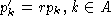, it is easily found that. Clearly. Hence, the normalizing factor and the a priori expectation of gap frequency is directly related and the same when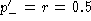. Using this relation one can readily write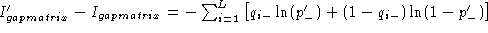so whenthe difference is equal to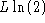, and the same as above is obtained. The result becomes independent of. The interpretation of a gap a priori probability of 0.5 also makes sense. If no prior knowledge is known about the alignment it makes sense to expect gaps to appear randomly, i.e. having an a priori expectation of 0.5. So a ``renormalization'' of the two sets of probabilities, the set of base frequencies, and the gap probability, ensures that the background probabilities sum to one and fulfill the formal claim to define information content.

The current version of Inform computes the information content according to, but MatrixPlot contains an option ``neg'' for which the baseline can be moved as described (when neg=n). Otherwise (when neg=y) the baseline is placed so that ``negative information content'' can show up on the profile. This can be useful in identifying positions which neither contains many or few gaps, but really is degenerated.

• Mutual information only for basepairs
As has been discussed by Gorodkin et al. (Comput. Appl. Biosci., 13:583-586, 1997) and used in the display of structure logos the mutual information content for RNA sequences can be limited to include only pairs of bases that are complementary. In that way a more relevant measure is constructed. This is done by defining a complementary matrix (Gorodkin et al. Nucl. Acids. Res. 25:3724-3732,1997) which lists the bases that are complementary. The complementary matrix lists all bases against themselves, and a number is assigned to indicate the degree of ``belief'' in the complementarity. The matrix is clearly symmetric and most positions in the matrix hold the value zero. The mutual information between position i and j in the alignment using the complementary matrix is given by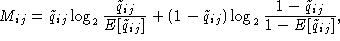(A)
where, A the alphabet, and where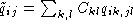and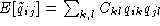. The element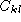of the complementary matrix lists the degree of complementarity between base k and l. The fraction of pairs of base k at position i and base l at position j is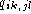and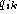is the fraction of base k at position i. Gaps is dealt with by having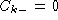for any base k. There are in particular two qualitative differences between this measure and the standard form of mutual information given by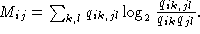(B)
First in (A) the observed and expected values are computed independently for two positions i and j, and the the overall covariance is computed. In contrast in (B) the observed and expected values are compared for each combination of the involved symbols. Secondly, in (A) only symbols for which a basepair rule is defined are included in the sum. In (B) all symbols are included (also gaps) even if it is already known that they do not (for RNA secondary structure) contribute to the covariance. This fact makes (A) much more robust to sampling size noise as illustrated below by two examples. In the Inform program measure (A) is referred to as mtype 2, and (B) to mtype 1. The upper triangle is mutual information content computed by type 2, and the lower triangle is the mutual information content computed by using type 1.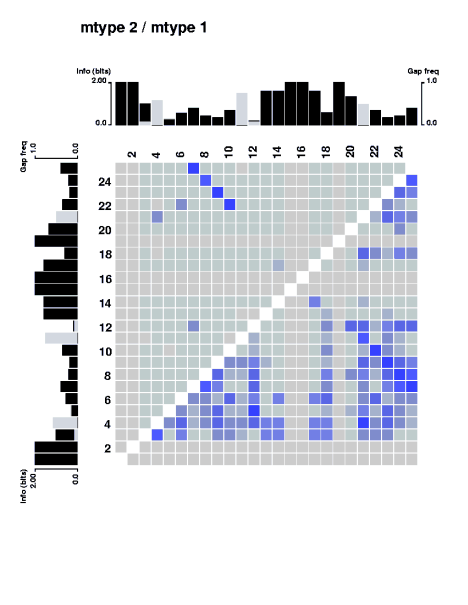Sample size corrections for plain sequence information content (e.g. Schneider et al. J. Mol. Biol. 188:415-431, 1986; Basharin, Theory Probability Appl. 4:333-336, 1959), and for the standard form of mutual information and correlation functions (e.g. Weiss and Herzel, J. Theor. Biol. 190:341-353, 1998) has previously been studied.

## Acknowledgements

Thanks to Anders Krogh for his contribution in the discussion of information content. Thanks to Claus A. Andersen, Lars J. Jensen, and Christopher Workman for suggestions and feedback.

## Reference

MatrixPlot: visualizing sequence constraints. J. Gorodkin, H. H. Stærfeldt, O. Lund, and S. Brunak. Bioinformatics. 15:769-770, 1999. (http://www.cbs.dtu.dk/services/MatrixPlot/)